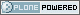# Neuroimaging Informatics Technology Initiative

##### Personal tools
You are here: Home » pixdim.html

# pixdim.html

## pixdim

```     pixdim[i] = voxel width along dimension #i, i=1..dim (positive)
- cf. ORIENTATION section below for use of pixdim
- the units of pixdim can be specified with the xyzt_units
field (also described far below).

/---------------------------------------------------------------------------/
/* UNITS OF SPATIAL AND TEMPORAL DIMENSIONS:
----------------------------------------
The codes below can be used in xyzt_units to indicate the units of pixdim.
As noted earlier, dimensions 1,2,3 are for x,y,z; dimension 4 is for
time (t).
- If dim=1 or dim < 4, there is no time axis.
- A single time series (no space) would be specified with
- dim = 4 (for scalar data) or dim = 5 (for vector data)
- dim = dim = dim = 1
- dim = number of time points
- pixdim = time step
- xyzt_units indicates units of pixdim
- dim = number of values stored at each time point   Bits 0..2 of xyzt_units specify the units of pixdim[1..3]
(e.g., spatial units are values 1..7).
Bits 3..5 of xyzt_units specify the units of pixdim
(e.g., temporal units are multiples of 8).
The toffset field can be used to indicate a nonzero start point for
the time axis.  That is, time point #m is at t=toffset+m*pixdim
for m=0..dim-1.
ORIENTATION

B.: Method 2 uses a factor `qfac` which is either -1 or 1; qfac is
stored in the otherwise unused pixdim.  If pixdim=0.0 (which
should not occur), we take qfac=1.  Of course, pixdim is only used

```

Created by Alex Clark